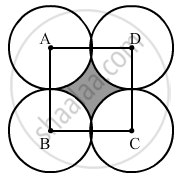Advertisement Remove all ads

# Four Equal Circles, Each of Radius 5 Cm, Touch Each Other, as Shown in the Figure. Find the Area Included Between Them. - Mathematics

Sum

Four equal circles, each of radius 5 cm, touch each other, as shown in the figure. Find the area included between them.Advertisement Remove all ads

#### SolutionRadius = 5 cm

AB = BC = CD = AD = 10 cm

All sides are equal, so it is a square.

Area of a square = Side2

Area of the square = 102  = 100 cm2

Area of the quadrant of one circle = 1/4 pi"r"^2

= 1/4xx22/7xx5xx5

= 19.64 cm

Area of the quadrants of four circles = 19.64 × 4 = 78.57 cm2

Area of the shaded portion = Area of the square - Area of the quadrants of four circles

= 100 - 78.57 cm2

= 21.43 cm2

Is there an error in this question or solution?
Advertisement Remove all ads

#### APPEARS IN

RS Aggarwal Secondary School Class 10 Maths
Chapter 18 Area of Circle, Sector and Segment
Exercise 18B | Q 37 | Page 833
Advertisement Remove all ads

#### Video TutorialsVIEW ALL 

Advertisement Remove all ads
Share
Notifications

View all notifications

Forgot password?
Course